SLUSBC8C December   2013  – July 2018

PRODUCTION DATA.

1. Features
2. Applications
3. Description
4. Revision History
5. Device Comparison Table
6. Pin Configuration and Functions
7. Specifications
8. Detailed Description
9. Application and Implementation
1. 9.1 Application Information
2. 9.2 Typical Applications
1. 9.2.1 bq51003 Wireless Power Receiver Used as a Power Supply
2. 9.2.2 Dual Power Path: Wireless Power and DC Input
10. 10Power Supply Recommendations
11. 11Layout
12. 12Device and Documentation Support
13. 13Mechanical, Packaging, and Orderable Information

• YFP|28

#### 8.3.13 Temperature Sense Resistor Network (TS)

bq51003 includes a ratiometric external temperature sense function. The temperature sense function has two ratiometric thresholds which represent a hot and cold condition. TI recommends an external temperature sensor to provide safe operating conditions for the receiver product. This pin is best used for monitoring the surface that can be exposed to the end user (that is, place the NTC resistor closest to the user).

Figure 28 allows for any NTC resistor to be used with the given VHOT and VCOLD thresholds.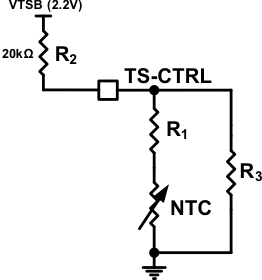Figure 28. NTC Circuit Used for Safe Operation of the Wireless Receiver Power Supply

The resistors R1 and R3 can be solved by resolving the system of equations at the desired temperature thresholds (see Equation 3 and Equation 4).

Equation 3.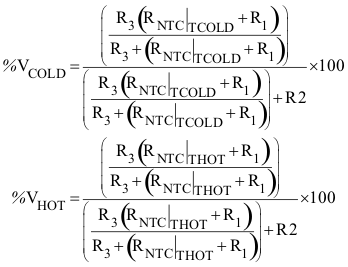Equation 4.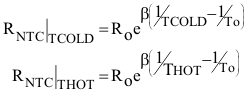where

• TCOLD and THOT are the desired temperature thresholds in degrees Kelvin
• RO is the nominal resistance
• and β is the temperature coefficient of the NTC resistor
• RO is fixed at 20 kΩ

An example solution is provided:

• R1 = 4.23 kΩ
• R3 = 66.8 kΩ

where the chosen parameters are:

• %VHOT = 19.6%
• %VCOLD = 58.7%
• TCOLD = –10°C
• THOT = 100°C
• β = 3380
• RO = 10 kΩ

Figure 29 shows the plot of the percent VTSB vs temperature.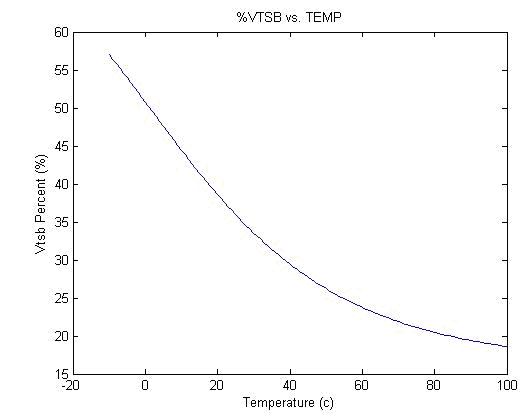Figure 29. Example Solution for an NTC resistor with RO = 10 kΩ and β = 4500

Figure 30 illustrates the periodic biasing scheme used for measuring the TS state. The TS_READ signal enables the TS bias voltage for 24 ms. During this period the TS comparators are read (each comparator has a 10-ms deglitch) and appropriate action is taken based on the temperature measurement. After this 24-ms period has elapsed, the TS_READ signal goes low, which causes the TS-Bias pin to become high impedance. During the next 35 ms (priority packet period) or 235 ms (standard packet period), the TS voltage is monitored and compared to 100 mV. If the TS voltage is greater than 100 mV then a secondary device is driving the TS-CTRL pin and a CTRL = ‘1’ is detected.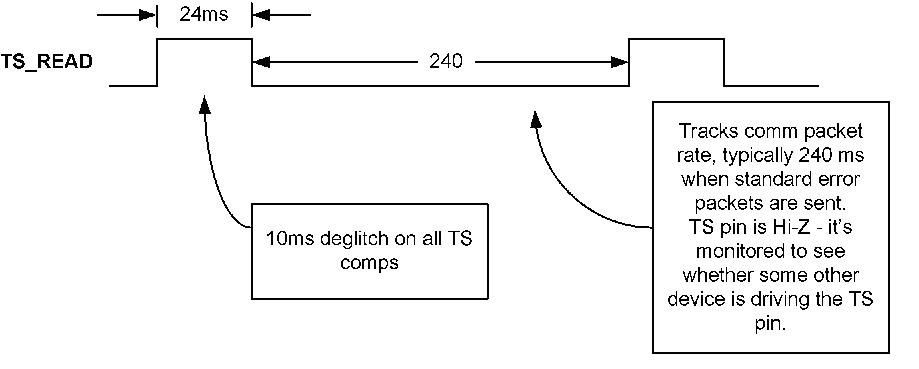Figure 30. Timing Diagram for TS Detection Circuit